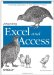# 5.4. Referencing Sheets, Ranges, and Cells

 < Day Day Up >

When you use Access VBA to automate Excel, there are some simple ways to refer to Excel objects. In addition, there are easy ways to cycle through the objects. Let's begin with Sheets.

#### 5.4.1. Working with Sheets

When you think about sheets in Excel, you generally talk about worksheets. However, there is another sheet object called a chart sheet. Each Excel worksheet is a member of two collections: the Sheets collection and the Worksheets collection. Both collections can be searched. Generally, I use the Sheets collection so that I use the same collection for all sheets in the application. However, if you cycle through the workbook and want to format regular worksheets a particular way, going through the Worksheets collection is your best bet. The chart sheets are in the Charts collection of the workbook object. Assuming that you have a variable called xlWs defined as an Excel.Worksheet, there are three basic ways to set the variable:

• Set xlWs = ActiveSheet

• Set xlWs = xlWb.Worksheets("Sheet1")

• Set xlWs = xlWb.Sheets("Sheet1")

Assuming that you just opened the workbook and Sheet1 is the active worksheet, any of these three methods will work. You can also cycle through the collection with the For Each...Next loop. For example, to print the name of each worksheet into the debug window, write:

`     For each xlWs in xlWb.Worksheets       Debug.Print xlWs.Name     Next xlWs `

This example assumes that you already have a reference to a workbook with a variable xlWb and that the variable xlWs refers to an Excel.Worksheet object. You can also refer to a worksheet by number, but I don't recommend it because the numbers are not constant if you move sheets around.

#### 5.4.2. Working with Ranges

Ranges can refer to a single cell, a group of contiguous cells, a group of non-adjacent cells, or every cell on a worksheet(s). The interesting thing about working with ranges is that many other objects are based on the Range type. For example, a cell is a range. A range is also a collection of cells. If you wanted to look at every individual cell in a range, you could use a For Each...Next loop, as shown in Example 5-19.

##### Example 5-19. Working with ranges example
` Public Sub CycleRange( ) Dim xlws As Worksheet Set xlws = Sheets("Sheet1") Dim xlrng As Excel.Range Dim xlrng2 As Excel.Range Set xlrng = xlws.Range("A1:B10") For Each xlrng2 In xlrng.Cells   Debug.Print "R" & xlrng2.Row & " - C" & xlrng2.Column Next xlrng2 Set xlrng2 = Nothing Set xlrng = Nothing Set xlws = Nothing End Sub `

If you run this loop from Excel VBA, you can see that the distinct ranges in the Cells collection are individual cells , since two combinations print in the debug window. This is useful when automating Excel from Access because you can check all of the cells in a range without knowing where the range is. For example, if there were a named range called ProductData, you could set a range equal to xlws.Range("ProductData") and cycle through the ranges in that range's .Cells collection.

#### 5.4.3. Working with Cells

Use the .Cells property of the worksheet object to return an individual cell based on the row and column number. In addition, when writing formulas, either refer to a cell as a string with A1 notation, using .Range with the worksheet object, or use R1C1 notation. Finally, as in Example 5-8, you can use the Offset function to return a cell reference that was offset a certain number of rows and columns from a particular cell.

Cells are the smallest data-holding object on an Excel worksheet available from the user interface. It is important to understand how to refer to each object type, particularly from Access, to make automation tasks easier.

 < Day Day Up >Integrating Excel and Access
ISBN: 0596009739
EAN: 2147483647
Year: 2005
Pages: 132

Similar book on Amazon